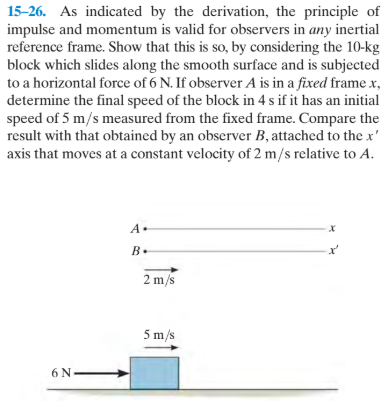# 15-26. As indicated by the derivation, the principle of impulse and momentum is valid for observers in any inertial reference frame. Show that this is so, by considering the 10-kg block which slides along the smooth surface and is subjected to a horizontal force of 6 N. If observer A is in a fixed framex, determine the final speed of the block in 4 s if it has an initial speed of 5 m/s measured from the fixed frame. Compare the result with that obtained by an observer B, attached to the x' axis that moves at a constant velocity of 2 m/s relative to A. A. B. 2 m/s 5 m/s 6 N

Questionhelp_outlineImage Transcriptionclose15-26. As indicated by the derivation, the principle of impulse and momentum is valid for observers in any inertial reference frame. Show that this is so, by considering the 10-kg block which slides along the smooth surface and is subjected to a horizontal force of 6 N. If observer A is in a fixed framex, determine the final speed of the block in 4 s if it has an initial speed of 5 m/s measured from the fixed frame. Compare the result with that obtained by an observer B, attached to the x' axis that moves at a constant velocity of 2 m/s relative to A. A. B. 2 m/s 5 m/s 6 N fullscreen

### Want to see this answer and more?

Experts are waiting 24/7 to provide step-by-step solutions in as fast as 30 minutes!*

*Response times may vary by subject and question complexity. Median response time is 34 minutes for paid subscribers and may be longer for promotional offers.
Tagged in
Science
Physics

### Other